# 5 Advanced Stats Techniques & When to Use Them

Jeff Sauro, PhDTo answer most user-research questions fundamental statistical techniques like confidence intervals, t-tests, and 2 proportion tests will do the trick.

But to answer some questions most effectively you need to use more advanced techniques.

Each of these techniques requires specialized software (e.g., SPSS, Minitab, R) and training on how to set up and interpret the results. But even if you aren’t ready to execute these techniques yourself, you can still learn what they are, when to use them, and some gotchas to look out for.

##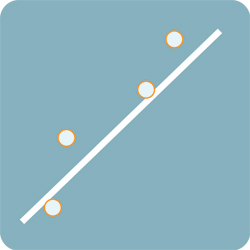When you want to understand what combination of variables best predicts a continuous outcome variable like customer satisfaction, likelihood to recommend, time on task, or attitudes toward usability, use regression analysis. This technique also goes by key-drivers analysis because you’re able to determine which independent variables have the biggest impact on your dependent (outcome) variable.

You can use both continuous and discrete variables (dummy coded) as independent (predictor) variables. For example, we found that usability for education software had the biggest relative impact on likelihood to recommend (NPS) using a multiple regression analysis. Regression analysis is a workhorse of statistical techniques and forms the basis of other methods for identifying optimal combinations of variables such as conjoint analysis.

Gotchas: Be sure a linear relationship exists between your variables by graphing them. You also don’t want the independent variables to correlate highly with each other (usually r > .8)—a condition called multicollinearity, which renders the regression equation unreliable.

##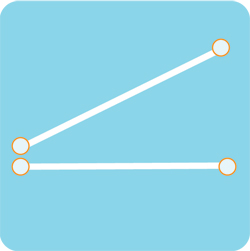An Analysis of Variance (ANOVA) tells you whether the means from more than two groups have a significant difference, such as the SUPR-Q scores across five websites. The more familiar t-test is a special case of the ANOVA when there are only two groups to compare.

What makes an ANOVA powerful though is it allows you to look at multiple variables at a time AND understand what combination results in the largest difference. This is called the interaction effect.

For example, you may be interested to know which form design users can complete more quickly: a form on one page, or the form split across two pages. That’s one variable (the form design) with two levels (form A and form B). This research question can be answered using a t-test. But if you want to understand how another variable, say the device type (mobile versus desktop), affects form completion time, you would use an ANOVA. It allows you to ensure that the form design that’s fastest for desktop completion isn’t a detriment to form completion on the mobile screen.

If you think this sounds similar to regression analysis, you’d be right. The ANOVA is just a regression analysis with discrete independent variables. Both techniques are part of a larger technique called General Linear Modeling (GLM), which we explain in detail in Chapter 10 of our forthcoming 2nd Edition of Quantifying the User Experience.

Gotchas: When you compare many groups, you increase the chance of finding a difference from chance alone (called alpha inflation). You should plan to use a multiple comparison technique, such as a Bonferroni adjustment or my preference, the Benjamini–Hochberg procedure, to differentiate the signal from the noise.

##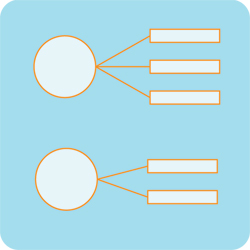Sometimes the variables you’re most interested in are those you can’t directly observe or measure easily. For example, there isn’t a direct measure of usability (no usability thermometer); instead, you have to rely on the outcomes of good and bad experiences to quantify usability. Factor analysis is a technique that takes many observed correlated variables and reduces them to a few latent (hidden) variables called factors.

For example, we used factor analysis[pdf] to identify usability as a single factor from the multiple correlated variables of task-time, completion rates, errors, and perceived task difficulty.

Factor analysis is also a staple of questionnaire development where you determine which items group together to best embody a construct. I used factor analysis extensively to build the SUPR-Q where I uncovered the latent variables of usability, loyalty, trust, and appearance from dozens of potential items.

Gotchas: There are a number of ways to determine how many factors are in your data and different researchers examining the same data may uncover a different number of factors. You generally need a large sample size to conduct a factor analysis and like many of the techniques, the relationship between variables should be linear.

##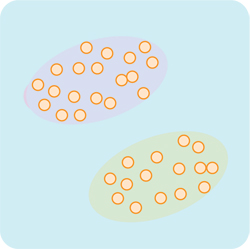When you want to know what items to group together you use cluster analysis. Like factor analysis, these groupings aren’t directly measurable but instead inferred from the data (another type of latent variable). Cluster analysis is the approach used in card sorting when you want to know how closely products, content, or functions relate from the users’ perspective.

Cluster analysis is also the technique we use to segment customers and build personas. When we conduct segmentation studies for clients we’ll look at a number of variables demographic (income, age) and psychographic (e.g. likelihood to use a new service) to see what defines a segment.

Gotchas: Like factor analysis, there’s some subjectivity involved in determining the number of clusters. You can easily justify more granular or more general clusters based on how closely you want the items to relate.

##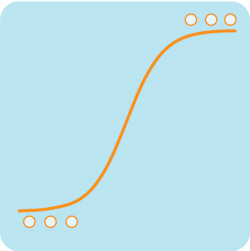It’s often the case that dependent variables are discrete and not continuous. For example, you may be primarily interested in purchase rates (purchase versus not purchase) or conversion rates (recommend, didn’t recommend); both are discrete binary.

In such cases, regular regression analysis won’t work. Instead, you’d use a different, but related technique, called logistic regression analysis, which converts the data using a logit transformation. In logistic regression, you still want to know what combination of independent variables best predicts the outcome, as in regular regression–the only difference is the outcome variable is discrete (usually binary).

For example, we’ve used logistic regression to understand how attitudes toward a service experience (favorable or unfavorable) and customer tenure (new versus existing) affects likelihood to repurchase.

Gotchas: While logistic regression doesn’t have the same linearity assumptions as regular regression, you still need to look for highly correlated independent variables (multicollinearity), need a large sample size, and interpret log-odd ratios, which is more challenging.

## Summary

Simple statistical techniques can answer many questions posed to UX researchers. There are times however when you need more advanced techniques to best answer the question.

Here’s how to differentiate between the five techniques based on their focuses, example research questions, and gotchas to watch out for.

 Technique Focus Research Example Gotcha Regression Analysis Best combination of variables to predict continuous outcome variables What features best predict likelihood to recommend? Correlated independent variables and linearity ANOVA Comparison of multiple variables plus interactions How does device type and form type affect task completion time? Alpha inflation Factor Analysis Identify latent variables that form groups (factors) What combination of items describes the constructs of appearance and trust? Subjective factors & linearity Cluster Analysis Uncover how items form latent groups Do users group two products together? Subjective clusters Logistic Regression Best combination of variables to predict discrete outcome variables How much does a service experience and tenure affect purchase rates? Correlated independent variables and linearity

0
0